## Solving the Moisture and Carbon-Nitrogen Equations Simultaneously

Tom Richard

In high school algebra we learn that for any number of independent equations we can usually solve for that same number of unknowns. In this case we have two equations (one for moisture and one for the carbon-nitrogen ratio), and we can solve them for any two unknowns. Normally we use this approach to develop a mix ratio of several different ingredients, knowing the moisture, carbon, and nitrogen contents of each. If we specify the quantities of all but two ingredients, and the C/N and moisture content we'd like to achieve in the mixture, we can solve for those two remaining quantities to get the mix we want.

In selecting which material quantities to specify and which to solve for as unknowns, it is important to use a little common sense. If your moisture goal is 60%, and you are trying to compost wet leaves, sawdust, grass, and food scraps, it would be smart to make sawdust one of the unknown quantities, since all the other materials are likely to have moisture contents greater than 60%. There is no way to bring the moisture content of a mix down by adding more of a wet ingredient, and, similarly, there is no way to bring the C/N ratio up by adding high nitrogen materials.

Another useful tip, particularly for dry ingredients, is to include water as one of the unknowns. Water will bring up the moisture content without altering the C/N ratio. And since water is cheap and usually readily available, it can be an easy way to develop an appropriate mix.

The solution can be obtained in a number of ways using linear algebra or matrices. With patience, one can use simple algebraic methods to solve the moisture equation for one of the unknown quantities, and then substitute that value in the C/N equation and solve the C/N equation for the other unknown. At that point, back-substitution into the solution of the moisture equation gives both unknowns in terms of known values.

The algebraic manipulations required for a mixture of three materials are fairly straightforward but do take a little time, as is evident from the solution below.

The three ingredient equation for moisture is: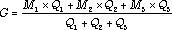```

in which:

Qn = mass of material n ("as is", or "wet weight")
G = moisture goal (%)
Mn = moisture content (%) of material n```

and the three ingredient equation for C/N ratio is:```

in which:

R = goal (C/N ratio)		Cn = carbon (%)
Nn = nitrogen (%)
and Mn and Qn are as previously defined

```

The resulting solutions are: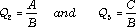where:

A = Q1 (M1 C3 (100 - M3 ) - M1 R N3 (100 - M3 ) - M3 C1 (100 - M1 )
+ R N3 (100 - M3 )G - R N1 (100 - M1 )G +C1 (100 - M1 )G
- C3 (100 - M3 )G +M3 R N1 (100 - M1 ))

B = R N2 (100 - M2 )G - R N2 (100 - M2 ) M3 - R N3 (100 - M3 )G
+ R N3 (100 - M3 )M2 - C2 (100 - M2 )G + C2 (100 - M2 )M3
+ C3 (100 - M3 )G - C3 (100 - M3 )M2

C = Q1 (R N1 (100 - M1 ) G - R N1 (100 - M1 ) M2 -R N2 (100 - M2 ) G
+ R N2 (100 - M2 ) M1 - C1 (100 -M1 ) G + C1 (100 - M1 ) M2
+ C2 (100 - M2 )G - C2 (100 - M2 ) M1 )

To see how this equation works, plug in the material characteristics from our previous example with grass and leaves, and the food scrap characteristics given below. Then solve for the quantity of leaves and/or food scraps needed to optimize C/N and moisture for 10 kg of grass.

 Ingredient Characteristics: Grass: Q1 = 10 M1 = 77% H2O C1 = 45% carbon N1 = 2.4% nitrogen Leaves: Q2 = ? M2 = 35% H2O C2 = 50% carbon N3 = 0.75% nitrogen Food scraps: Q3 = ? M3 = 80% H2O C3 = 42% carbon N3 = 5.0% nitrogen Mixture Goals: Moisture: G = 60% C/N ratio: R = 30
A = 10 x (77 x 42 x (100 - 80) - 77 x 30 x 5.0 x (100 - 80) - 80 x 45 x (100 - 77)
+ 30 x 5.0 x (100 - 80) x 60 - 30 x 2.4 x (100 - 77) x 60 +45 x (100 - 77) x 60
- 42 x (100 - 80) x 60 +80 x 30 x 2.4 x (100 - 77))

A = -243,000

B = 30 x 0.75 x (100 - 35) x 60 - 30 x 0.75 x (100 - 35) x 80 - 30 x 5.0 x (100 - 80) x 60
+ 30 x 5.0 x (100 - 80) x 35 - 50 x (100 - 35) x 60 + 50 x (100 - 35) x 80
+ 42 x (100 - 80) x 60 - 42 x (100 - 80) x 35

B = - 18,250

C = 10 x (30 x 2.4 x (100 - 77) x 60 - 30 x 2.4 x (100 - 77) x 35
- 30 x 0.75 x (100 - 35) x 60 + 30 x 0.75 x (100 - 35) x 77 - 45 x (100 - 77) x 60
+ 45 x (100 - 77) x 35 + 50 x (100 - 35) x 60 - 50 x (100 - 35) x 77 )

C = -148,625

Remembering thatwe find that:

Q2 = 13.31 kg and Q3 = 8.14 kg

Thus if we mix 13 kg of leaves and 8 kg of food scraps with the initial 10 kg grass clippings, the mixture will achieve our goals of 60% moisture and a 30:1 C/N ratio.

Note that this simultaneous solution for three ingredients depends entirely on having the right three ingredients to combine. With many combinations the resulting Q2 and/or Q3 will be negative, indicating that no solution is possible. In that case you can add an additional material to add to the mix, such as sawdust or wood chips if the moisture or nitrogen levels are too high. Of course, if we add more ingredients, we also need a different formula to determine the solution.

For increasing numbers of materials, this formula becomes even more complicated. The solution for a mixture of four ingredients follows.

The four ingredient equation for moisture is: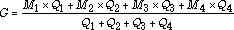and the four ingredient equation for C/N ratio is: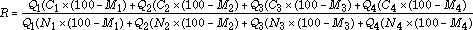where all terms are as previously defined

If we know the carbon, nitrogen, and moisture contents of each of these materials, specify goals for moisture and C/N ratio of the mixture, and quantities of Q1 and Q2, then we can solve for Q3 and Q4. The solution is:andwhere

D= -(Q1C4(100-M4)G+Q2C4(100-M4)G-Q2C2(100-M2)G-Q1C1(100-M1)G
-Q1RN4(100-M4)G-Q2RN4(100-M4)G+RQ1N1(100-M1)G+RQ2N2(100-M2)G
-M4RQ1N1(100-M1)-M1Q1C4(100-M4)+M4Q1C1(100-M1)-M2Q2C4(100-M4)
-M4RQ2N2(100-M2)+M1Q1RN4(100-M4)+M4Q2C2(100-M2)+M2Q2RN4(100-M4))

E= RN3(100-M3)G-RN3(100-M3)M4-C3(100-M3)G+C3(100-M3)M4-RN4(100-M4)G
+RN4(100-M4)M3+C4(100-M4)G-C4(100-M4)M3

and

F = -RN3(100-M3)GQ1-RN3(100-M3)GQ2+RN3(100-M3)M1Q1
+RN3(100-M3)M2Q2+C3(100-M3)GQ1+C3(100-M3)GQ2
-C3(100-M3)M1Q1-C3(100-M3)M2Q2+RQ1N1(100-M1)G
-RQ1N1(100-M1)M3+RQ2N2(100-M2)G-RQ2N2(100-M2)M3
-Q1C1(100-M1)G+Q1C1(100-M1)M3-Q2C2(100-M2)G+Q2C2(100-M2)M3

This is where computers come in handy. These simultaneous solutions are included on spreadsheets you can download and use on your own computer.

Acknowledgement:  Helpful reviews of this document and the accompanying spreadsheet were provided by Nancy Trautmann.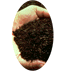Cornell Composting Science & Engineering Composting in Schools Resources

Cornell Waste Management Institute © 1996
Cornell University
Ithaca, NY 14853-5601
607-255-1187